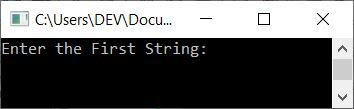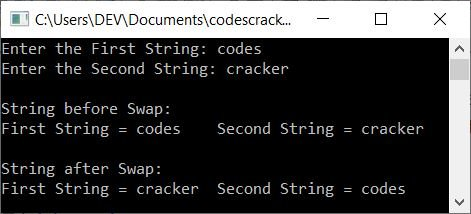# C++ program to swap two strings

In this article, you will learn and get code to swap two strings entered by the user using a C++ program. Here is the list of programs that can swap two given strings:

## In C++, use the library function to swap two strings

To swap two strings in C++ programming, you have to ask the user to enter the two strings. The value of both strings gets stored in two variables, say, strOne (the first string) and strTwo (the second string). Now swap these two strings using a third variable, say strTmp, with the help of the library function strcpy(), as shown in the program given below:

```#include<iostream>
#include<stdio.h>
#include<string.h>
using namespace std;
int main()
{
char strOne, strTwo, strTmp;
cout<<"Enter the First String: ";
gets(strOne);
cout<<"Enter the Second String: ";
gets(strTwo);
cout<<"\nString before Swap:\n";
cout<<"First String = "<<strOne<<"\tSecond String = "<<strTwo;
strcpy(strTmp, strOne);
strcpy(strOne, strTwo);
strcpy(strTwo, strTmp);
cout<<"\n\nString after Swap:\n";
cout<<"First String = "<<strOne<<"\tSecond String = "<<strTwo;
cout<<endl;
return 0;
}```

This program was built and runs under the Code::Blocks IDE. Here is its sample run:Now supply two strings, say codes as the first and cracker as the second string. Here is the sample run with these two strings as input:The following block of code:

```strcpy(strTmp, strOne);
strcpy(strOne, strTwo);
strcpy(strTwo, strTmp);```

is responsible for swapping two strings. For example, if the user enters the same two strings as given in the above program's sample run. Initially, strOne=codes and strTwo=cracker. Now, after executing the first statement (of the three lines of code listed above):

`strcpy(strTmp, strOne);`

The value of strOne gets copied to strTmp. So strTmp=codes. In a similar way, after executing the following (second) statement:

`strcpy(strOne, strTwo);`

The value of strTwo gets copied to strOne. So strOne=cracker. And using the third statement, that is:

`strcpy(strTwo, strTmp);`

The value of strTmp gets copied to strTwo. So strTwo=codes. In this way, swapping gets performed using the strcpy() library function in C++.

## Swap two strings without using a library function in C++

This program just copies the string manually (using user-based code) without using the library function strcpy():

```#include<iostream>
#include<stdio.h>
using namespace std;
int main()
{
char strOne, strTwo, strTmp;
int i=0;
cout<<"Enter the First String: ";
gets(strOne);
cout<<"Enter the Second String: ";
gets(strTwo);
cout<<"\nString before Swap:\n";
cout<<"First String = "<<strOne<<"\tSecond String = "<<strTwo;
while(strOne[i]!='\0')
{
strTmp[i] = strOne[i];
i++;
}
strTmp[i] = '\0';
i=0;
while(strTwo[i]!='\0')
{
strOne[i] = strTwo[i];
i++;
}
strOne[i] = '\0';
i=0;
while(strTmp[i]!='\0')
{
strTwo[i] = strTmp[i];
i++;
}
strTwo[i] = '\0';
cout<<"\n\nString after Swap:\n";
cout<<"First String = "<<strOne<<"\tSecond String = "<<strTwo;
cout<<endl;
return 0;
}```

This program produces the same output as the previous program. In the above program, instead of using the strcpy() function to copy strings, we've done the same thing using user-based code.

Note: To learn more about copying one string to another, refer to the copy string program to get all the required information about the topic. There you will get full details about how the code given in the previous program works.

#### The same program in different languages

C++ Quiz

« Previous Program Next Program »